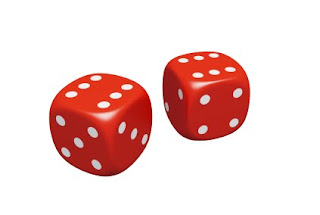### Valentine's Dice GameThis dice game is a variation of my absolute favorite dice game to practice multiplication facts- Circles and Stars. For Valentine's math, I call the game X's & O's since those letters represent hugs and kisses.

Roll one die and draw that many O's on your paper. Roll another die and draw that many X's on the inside of each circle. Write out the multiplication sentence to go with that problem. Have your child count the X's in each circle for the total. For example, two O's with three X's in each O equals six X's. (2 x 3 = 6) Take turns rolling the dice and illustrating the number sentences. I usually have each player roll ten times. Have your child add all the products (total amount of X's) each person has with a calculator to see who wins.

The example below has stars instead of X's.2 x 3 = 6